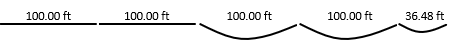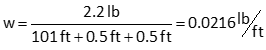## 4. Example Taping Corrections

### a. Information

A steel add tape was calibrated at 70°F with full support and found to be 100.004 feet long under 23 pounds of pull. Its weight and cross-sectional area were measured and recorded as 2.2 pounds and 0.004 sq in. The tape steel had a modulus of elasticity of 2.9x107 psi and thermal expansion coefficient of 6.5x10-6 ft/ft/°F.

The tape was used on a day when the temperature was 50°F. A distance was measured and recorded as 436.48 ft. The first two full tape segments were fully supported. The remainder of the line consisted of end only support segments: two 100 ft and one 36.48 ft, Figure E-16.Figure E-16Example 1

A tension handle was used to apply a consistent 15 pound pull for all measurements.

### b. What is the correct measured distance for the use conditions?

#### (1) Incorrect tape length

Set up and solve Equations E-1 and -2#### (2) Temperature

Set up and solve Equations E-5 and -6#### (3) Pull

Set up and solve Equations E-7 and -8#### (4) Sag

Determine the tape's weight per foot. The entire tape weighed 2.2 pounds. Because it's an add tape, it is 101 ft long. Also, at both ends of the tape is an additional half-foot.Use Equation E-3 to determine the sag error for a full tape lengthUse Equation E-3 to determine the sag error for a full the last partial lengthUse Equation E-4 to compute the total sag corrections#### (5) Corrected length

Add the corrections to the measured distance.